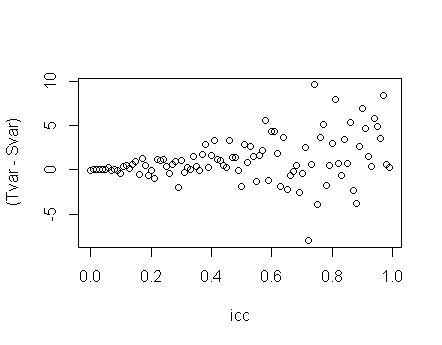top of page
Search
• Burak AYDIN

# Totalvariance=eij+u0j?

An empty two level model has 2 variance components, eij and u0j (icc=u0j/(u0j+eij)).

Is var(dependent variable)=eij+u0j ?Lets write a function;

require(nlme) require(MASS)

#ICCbreaks from 0 to .99, how many breaks for ICC?

# what is your total variance for a two level null model (eij+u0j)?

# on a scale 1 to 3 how unbalanced your data? 1 is perfect balance, 2 is some unbalance, 3 is ..

singleVSmlmVAR=function(ICCbreaks=100,totalvar=100,unbal=1){

if(ICCbreaks<3)ICCbreaks=3 plotdata=matrix(0,ncol=3,nrow=ICCbreaks)

icc=seq(0,.99,length.out=ICCbreaks) for(i in 1:ICCbreaks){ l1var=totalvar-(icc[i]*totalvar) l2var=(icc[i]*totalvar) if(unbal==3)probs=runif(50) if(unbal==3)wid=data.frame(l1id=1:1000,l2id=sample(1:50,1000,prob=runif(50)/sum(probs),replace = T),rij=mvrnorm(1000,0,Sigma=l1var,empirical = T)) if(unbal==1)wid=data.frame(l1id=1:1000,l2id=rep(1:50,each=20),rij=mvrnorm(1000,0,Sigma=l1var,empirical = T)) if(unbal==2)wid=data.frame(l1id=1:1000,l2id=sample(1:50,1000,replace = T),rij=mvrnorm(1000,0,Sigma=l1var,empirical = T))

cid=data.frame(l2id=1:50,u0j=mvrnorm(50,0,Sigma=l2var,empirical = T)) iccdata=merge(wid,cid,by="l2id") iccdata\$y=with(iccdata,100+rij+u0j) plotdata[i,1]=var(iccdata\$y) Null.Model<-lme( y~1,random=~1|l2id,data=iccdata) plotdata[i,2]=(as.numeric(VarCorr(Null.Model))+as.numeric(VarCorr(Null.Model))) plotdata[i,3]=icc[i] plotdata=data.frame(plotdata) names(plotdata)=c("Svar","Tvar","icc") } return(plotdata) }

1. Balanced case, 100 ICCs, eij+u0j=100

attach(singleVSmlmVAR(100,100,1)) plot(icc,(Tvar-Svar))

Tvar is eij+u0j and Svar=var(dependent variable)

The difference is 0 when ICC is 0 and only about 2 when ICC is 1 (compare to 100)2. Unbalanced case, 100 ICCs, eij+u0j=100

attach(singleVSmlmVAR(100,100,2)) plot(icc,(Tvar-Svar))

The difference is 0 when ICC is 0 and varies as ICC goes to 1, ranged between -10 and 102. Sparse case, 100 ICCs, eij+u0j=100

attach(singleVSmlmVAR(100,100,3)) plot(icc,(Tvar-Svar))

The difference is 0 when ICC is 0 and varies as ICC goes to 1, ranged between -30 and 30.